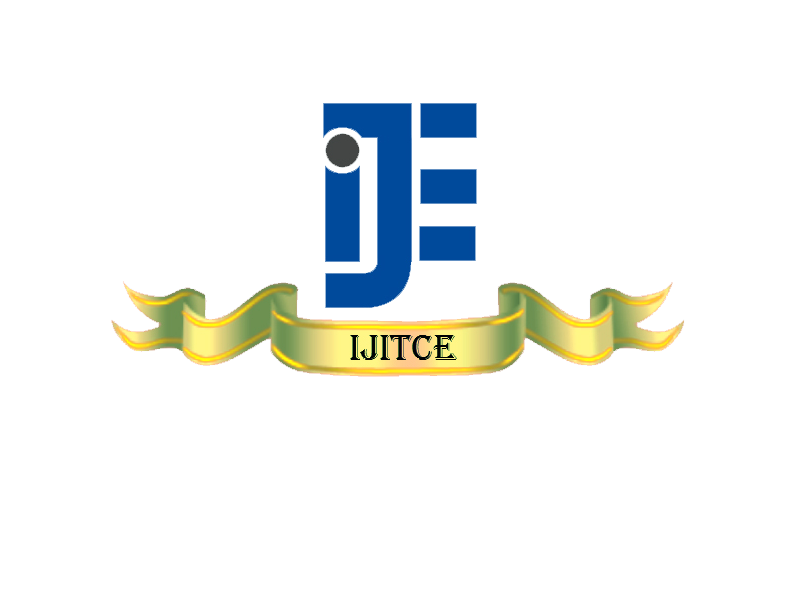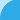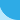# November 2012 Issue Vol.2 No.11

### Dirichlet series and approximate method for the solution of axisymmetric flow over a stretching sheet

Vishwanath B. Awati
Department of Mathematics,Rani Channamma University,Belagavi - 591156, India.

Abstract: Abstract— We study the boundary layer flows induced by the axisymmetric stretching of a sheets are studied using more suggestive schemes. The equation of motion of a axisymmetric flow over a stretching sheet and the sheet is stretched with a velocity is proportional to the distance from the vertical axis. The governing nonlinear differential equations are reduced to nonlinear linear ordinary differential equations (ODEs) by using similarity transformations. The resulting nonlinear ODEs are solved by using fast convergent Dirichlet series method and an approximate analytical method by Method of stretching of variables. These methods have advantages over pure numerical methods for obtaining the derived quantities accurately for various values of the parameters involved at a stretch and these are valid in much larger domain as compared with the classical numerical schemes.

Keywords: Axisymmetric flow; boundary layer equations; Stretching sheet; Dirichlet series; Powell’s method; Least square approximation

### Optimization of Tribological Parameters in Al6061/SiC Metal Matrix Composite by Taguchi’s Technique

Ashok Kr Mishra1, Rakesh Sheokand2, Gopal Pr Yadav3, Dr. B. C. Sharma4, Dr. R. K. Srivastava5
1. Astt. Prof. in BRCM College of Engineering & Technology, Bahal
2. Astt. Prof. in Jind Institute of Engineering & Technology, Jind
3. Prof. & Head Deptt. of Mechanical, BRCM CET, Bahal
4. Principal & Prof. in BRCM CET, Bahal
5. Prof. & Head Deptt. of Production, B. I. T. Sindri, Jharkhand

Abstract: Tribological behavior of aluminium alloy Al 6061 reinforced with silicon carbide (10%) fabricated by stir casting process was investigated. Dry sliding wear test was conducted to understand the tribological behavior of samples. This study is carried out to optimize the tribological properties: wear rate & frictional force of Al / SiC metal matrix composite. The experiments were conducted as the Taguchi design of experiment. A L9 orthogonal array was selected for analysis of the data. The wear parameters chosen for the experiment were: sliding speed, applied load & sliding distance. Each parameter was assigned three levels. Signal to Noise ratio analysis has been carried out to determine optimal parametric condition, which yields minimum wear rate & frictional force. Investigation to find the influence of applied load, sliding speed and sliding distance on wear rate, as well as the frictional force during wearing process was carried out using ANOVA and regression equations for each response were developed. Objective of the model was chosen as “smaller the better” characteristics to analyze the dry sliding wear resistance. Result show that sliding distance and load have the highest influence on minimum wear rate and frictional force respectively. Finally, confirmation tests were carried out to verify the experimental results and Scanning Electron Microscopic (SEM) studies were done on the wear surfaces.

Keywords: Metal matrix composites; Silicon Carbide; Taguchi Technique; Signal to Noise